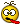Hello,

GDP per capita (USD); GDP Index (2012 = 100); Economic growth rate (%GDP); Consumption (% increase); Investment ( % change); Unemployment Rate; Government surplus (% of GDP); Public Savings (% of GDP); Money supply (annual increase in; Inflation Rate (CPI, % change); Central Bank Interest Rate (%); Stock Market index (% change); Net Exports (% of GDP); National Debt (% of GDP)

How to calculate change in V from the formula VM = P * Y? Is this even possible? If so, how would we do that?

Thanks,
I didn't find the right solution from the internet.
References: http://www.debate.org/forums/economics/

Animated Promotional Video Example